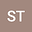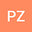loading page

Uniform convergent modified weak Galerkin method for convection-dominated two-point boundary value problems
••Peng Zhu
Jiaxing Vocational and Technical College
Author Profile## Abstract

In this paper, a modified weak Galerkin finite element method on Shishkin mesh has been developed and analyzed for the singularly perturbed convection-diffusion-reaction problems. The proposed method is based on the idea of replacing the standard gradient (derivative) and convection derivative by modified weak gradient (derivative) and modified weak convection derivative, respectively, over piecewise polynomials of degree $k\geq1$. The present method is parameter-free and has less degree of freedom compared to the weak Galerkin finite element method. Stability and convergence rate of $\mathcal {O}((N^{-1}\ln N)^k)$ in the energy norm are proved. The method is uniformly convergent, i.e., the results hold uniformly regardless of the value of the perturbation parameter. Numerical experiments confirm these theoretical findings on Shishkin meshes. The numerical examples are also carried out on B-S meshes to confirm the theoretical results. Moreover, the proposed method has the optimal order error estimates of $\mathcal {O}(N^{-(k+1)})$ in a discrete $L^2-$ norm and converges at superconvergence order of $\mathcal {O}((N^{-1}\ln N)^{2k})$ in the discrete $L_\infty-$ norm.